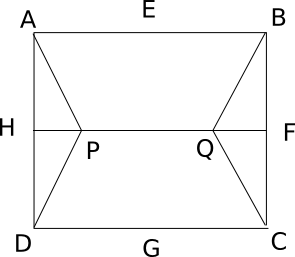Question 17

# Consider a square ABCD with midpoints E, F, G, H of AB, BC, CD and DA respectively. Let L denote the line passing through F and H. Consider points P and Q, on L and inside ABCD such that the angles APD and BQC both equal 120°. What is the ratio of the area of ABQCDP to the remaining area inside ABCD?

SolutionConsider side of square as 10 units. So HD=5 and HP=$$\frac{5}{\sqrt3}$$ . So now area of triangle HPD=$$\frac{12.5}{\sqrt3}$$. Also Area APD=Area BQC=2*AreaHPD=$$\frac{25}{\sqrt3}$$. So numerator of required answer is $$100-\frac{50}{\sqrt3}$$ and denominator as $$\frac{50}{\sqrt3}$$. Solving we get answer as $$2\sqrt{3}-1$$.